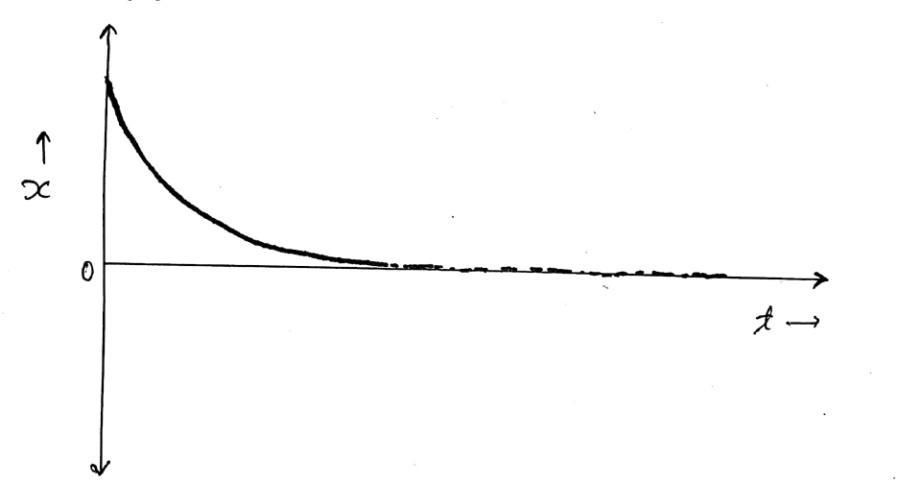## Critically Damped System (ξ = 1)Fig.1(Critically Damped System)

• Critically damped system(ξ=1): If the damping factor ξ is equal to one, or the damping coefficient c is equal to critical damping coefficient "cc", then the system is said to be a critically damped system.
$\xi=1 \quad \text OR \quad {c \over c_c} = 1\implies c = c_c$
• Two roots for critically damped system are given by S1 and S2 as below:
$S_1 = \big [-\xi + \sqrt{\xi^2 -1} \big] \omega_n \\ S_2 = \big [-\xi - \sqrt{\xi^2 -1} \big] \omega_n$
• For $ξ=1$; $S_1 = S_2 = -\omega_n$
Here both the roots are real and equal, so the solution to the differential equation can be given by
$x = (A + Bt)e^{-\omega_n t} \quad \quad ...(1)$
• Now differentiating equation (1) with respect to ‘t’, we get:
$\mathring x = Be^{-\omega_nt} - \omega_n(A + Bt)e^{-\omega_nt} \quad \quad ...(2)$
• Now, let at
$t = 0$ : $x = X_0$
$t = 0$ : $\mathring x =0$

• Substituting these values in equation (1):

$X_0 = A \quad ...(3)$
• Same way, from equation (2), we get
\begin{aligned} 0&= B - \omega_n(A + 0) \\ 0&= B - \omega_nA \\ B&= \omega_nA \\ B&= \omega_nX_0 \qquad ...(4) \end{aligned}
• Now putting the values of A and B in equation (1), we get:
\begin{aligned} x&= (X_0 + \omega_nX_0t)e^{-\omega_nt} \\ x&= X_0(1 + \omega_nt)e^{-\omega_nt} \qquad ...(5) \end{aligned}

### Conclusion

• From above equation (5), it is seen that as time t increases, the displacement x decreases exponentially.

• The motion of a critically damped system is aperiodic (aperiodic motion motions are those motions in which the motion does not repeat after a regular interval of time i.e non periodic motion) and so the system does not shows vibrations.

• For critically damped systems, if a system is displaced from its initial position, it will try to reach its mean position in a very short time.

• Critically damped systems are generally seen in hydraulic doors closer as it is necessary for the door to come to its initial position in a very short time.